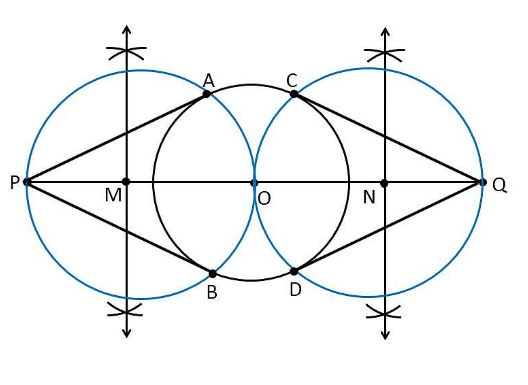# Draw a circle of radius 3 cm. Take two points P and Q on one of its extended diameter each at a distance of 7 cm from its centre. Draw tangents to the circle from these two points P and Q Give the justification of the construction

To construct a tangent from given conditions

1. Let us draw a circle with a radius of 3cm with centre “O”.

2. Draw a diameter of a circle and it extends 7 cm from the centre and marks it as P and Q.

3. Draw the perpendicular bisector of the line PO and mark the midpoint as M.

4. Draw a circle with M as centre and MO as the radius

5. Now join the points PA and PB in which the circle with radius MO intersects the circle of circle 3cm.

6. Now PA and PB are the required tangents.

7. Similarly, from point Q, we can draw the tangents.

8. From that, QC and QD are the required tangents.Justification

We have to prove that PQ and PR are the tangents to the circle of radius 3 cm with centre O.

To prove this, join OA and OB.

From the construction,

∠PAO is an angle in the semi-circle.

We know that angle in a semi-circle is a right angle, so it becomes,

∴ ∠PAO = 90°

Such that

⇒ OA ⊥ PA

Since OA is the radius of the circle with radius of 3 cm, PA must be a tangent of the circle.

Similarly, we can prove that PB, QC and QD are the tangents of the circle.